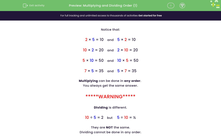# Understand the Order of Multiplication and Division Statements

In this worksheet, students will show understanding that multiplication can be done in any order, but division cannot.Key stage:  KS 1

Curriculum topic:   Number: Multiplication and Division

Curriculum subtopic:   Understand Order for Multiplication/Division

Difficulty level:#### Worksheet Overview

This activity is about the order of multiplying and dividing.

Notice that:

2 × 5 = 10    and    5 × 2 = 10

10 × 2 = 20    and    2 × 10 = 20

5 × 10 = 50    and    10 × 5 = 50

7 × 5 = 35    and    5 × 7 = 35

Multiplying can be done in any order

You always get the same answer.

*****WARNING*****

Dividing is different.

10 ÷ 5 = 2    but     5 ÷ 10 = ½

They are not the same.

Dividing cannot be done in any order.

When we are dividing, we usually put the biggest number first and divide that by one of the other numbers.The best way to get your head around this is to practise by doing some questions!

### What is EdPlace?

We're your National Curriculum aligned online education content provider helping each child succeed in English, maths and science from year 1 to GCSE. With an EdPlace account you’ll be able to track and measure progress, helping each child achieve their best. We build confidence and attainment by personalising each child’s learning at a level that suits them.

Get started